# Partitioning edge-connectivity

 Importance: Medium ✭✭
 Author(s): DeVos, Matt
 Subject: Graph Theory » Basic Graph Theory » » Connectivity
 Keywords: edge-coloring edge-connectivity
 Prize: bottle of wine (DeVos)
 Posted by: mdevos on: March 7th, 2007
Question   Letbe an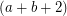-edge-connected graph. Does there exist a partitionof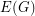so thatis-edge-connected andis-edge-connected?

By the Nash-Williams/Tutte theorem ([NW] or [T]) on disjoint spanning trees, the above conjecture is true ifis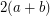-edge-connected. This is the only partial result I know of. Here is a related conjecture.

Conjecture   There exists a fixed integerso that every-edge-connected graphhas a subset of edgeswith the property that every edge-cut ofhas betweenandof its edges in.

The valuesandare of no special importance in the above conjecture. Indeed, an affirmative answer to the above problem withandreplaced byandfor any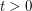would still be valuable - and in particular, would imply the 2+epsilon flow conjecture.

Definition: Letbe a graph and letbe a partition of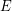. We say thatis-courteous if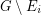is-edge-connected for every.

Problem   What is the smallest integerso that every 3-edge-connected graph has a 2-courteous coloring of size?

It is known (see [DJS]) that. It would be quite interesting if the truth were in fact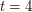. An improvement on the current upper bound would have some consequences for certain flow problems and cycle-cover problems. In general, one may define a functionso that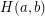is the smallest integer(orif none exists) so that every-edge-connected graph has a-courteous coloring of size. It is known (see [DJS]) that, and that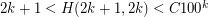. Two special cases when better values are known areand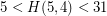.

## Bibliography

[DJS] M. DeVos, T. Johnson, P.D. Seymour, Cut-coloring and circuit covering

[Ed] J. Edmonds, Minimum Partition of a Matriod into Independent Subsets, J. Res. Nat. Bur. Standards 69B (1965) 67-72. MathSciNet

[NW] C.S.J.A. Nash-Williams, Edge Disjoint Spanning Trees of Finite Graphs, J. London Math. Soc. 36 (1961) 445-450. MathSciNet

[T] W.T. Tutte, On the problem of decomposing a graph into n connected factors, J. London Math. Soc. 36 (1961), 221-230. MathSciNet

* indicates original appearance(s) of problem.